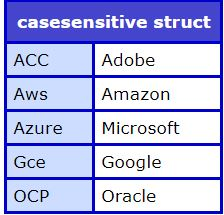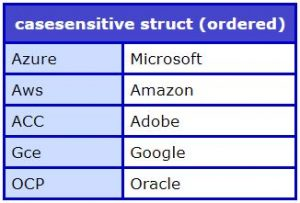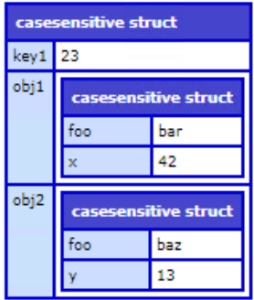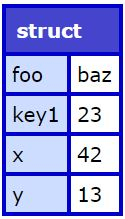Structs had been an Integral part of ColdFusion Markup Language. Using Structs we can define complex Objects and use it as such for complex operations. There have been so many functions that have been exposed for creating/manipulating and validating the Struct Objects.

ColdFusion has two types of Structs as follows:

• Normal Structs
• Ordered Structs

With Project Stratus (ColdFusion 2021) release, we are adding a capability for preserving the keys of Structs to avoid case-sensitivity issues.

A little bit background around the need comes from Project Stratus release which has so many Cloud related features and many of these features and functionality require the data and configuration to be stored in the form of Structs.

Earlier a developer has to use the

To avoid all this kind of configuration while writing some new code, a developer can use the case sensitive Structs, which automatically takes care of preserving the case of Struct Keys.

Lets go into the details of how to define a case sensitive structs:

Syntax:

`mystruct = new ``StructNew``(``"casesensitive"``);`

`//Implicit Notation`
` ``mystruct = \${key:value};`

`<``cfscript``>`
`cloudVendors=``StructNew``(``"casesensitive"``);`
`cloudVendors.Azure=``"Microsoft"``;`
`cloudVendors.Aws= ``"Amazon"``;`
`cloudVendors.ACC = ``"Adobe"``;`
`cloudVendors.Gce= ``"Google"``;`
`cloudVendors.OCP = ``"Oracle"`
`writeDump(cloudVendors);`
`</``cfscript``>`

The above Code will output as shown below:The implicit way of defining the case sensitive struct is to prepend a \$ token before the Struct Definition.
`<``cfscript``>`
`cloudVendors= \$ {`
`Azure : ``"Microsoft"``,`
`Aws :  ``"Amazon"``,`
`ACC : ``"Adobe"``,`
`Gce : ``"Google"``,`
`OCP : ``"Oracle"`
`}`
`writeDump(cloudVendors);`
`</``cfscript``>`

The case sensitivity aspect is also extended to Ordered Structs as shown below, For Ordered Structs, we just need to prepend \$ before the Ordered Struct Definition

`<``cfscript``>`
`    ``cloudVendors= \$ [`
`    ``Azure : ``"Microsoft"``,`
`    ``Aws :  ``"Amazon"``,`
`    ``ACC : ``"Adobe"``,`
`    ``Gce : ``"Google"``,`
`    ``OCP : ``"Oracle"`
`    ``];`
`    ``writeDump(cloudVendors);`
`</``cfscript``>`

The above Code will output as shown below:

`<!---------------------------------------------------`
`check the order of Insertion as well case-sensitivity`
`matters here.`The functional way of writing the same code is as shown below:

`<``cfscript``>`
`cloudVendors=``StructNew``(``"ordered-casesensitive"``);`
`cloudVendors.Azure=``"Microsoft"``;`
`cloudVendors.Aws= ``"Amazon"``;`
`cloudVendors.ACC = ``"Adobe"``;`
`cloudVendors.Gce= ``"Google"``;`
`cloudVendors.OCP = ``"Oracle"`
`writeDump(cloudVendors);`
`</``cfscript``>`

The Metadata for Structs have also been enhanced to add the case-sensitive information

`<``cfscript``>`

`// For CaseSensitive Structs`
`cloudVendors = \$[`
`Azure : ``"Microsoft"``,`
`Aws : ``"Amazon"``,`
`ACC : ``"Adobe"``,`
`Gce : ``"Google"``,`
`OCP :``"Oracle"`
`];`
`writeDump(cloudVendors.``getMetadata``());`

`// For Ordered CaseSensitive Structs`
`cloudVendors = \${`
`Azure : ``"Microsoft"``,`
`Aws : ``"Amazon"``,`
`ACC : ``"Adobe"``,`
`Gce : ``"Google"``,`
`OCP :``"Oracle"`
`};`

`writeDump(cloudVendors.``getMetadata``());`
`</``cfscript``>`

With this release we have also added a BIF to check whether the corresponding Struct Object is case-sensitive Struct or not.StructIsCaseSensitive(strctObj)

The corresponding member function for this is isCaseSensitive()

Lets take an example to check how we can use this function to get the required information.

`<``cfscript``>`
`cloudVendors = \$[`
`Azure : ``"Microsoft"``,`
`Aws : ``"Amazon"``,`
`ACC : ``"Adobe"``,`
`Gce : ``"Google"``,`
`OCP :``"Oracle"`
`];`

`writeOutput``(StructIsCaseSensitive(cloudVendors)); // should output YES`
`writeOutput``(cloudVendors.isCaseSensitive());// should output YES`
`writeOutput``(cloudVendors.isOrdered());// should output YES`
`</``cfscript``>`

Merging Structs:
Using the implicit notation we can easily merge two or more Struct Objects. Lets simulate it with an example
`<``cfscript``>`
`obj1 =\${ foo: ``'bar'``, x: 42 };`
`obj2 =\${ foo: ``'baz'``, y: 13 };`

`mergedObj = \${obj1, obj2, ``"key1"``:``"23"``};`
`writeDump(mergedObj);`
`</``cfscript``>`By using this functionality we can even override keys of the structs using the Spread Operator. Consider a scenario where a developer wants to merge data from multiple structs and override the keys which are same in multiple structs. This can be done by using Merging Structs Functionality using Spread Operator.

Lets simulate it with an example

`<``cfscript``>`
`obj1 =\${ foo: ``'bar'``, x: 42 };`
`obj2 =\${ foo: ``'baz'``, y: 13 };`
`mergedObj = {...obj1, ...obj2, ``"key1"``:``"23"``};`
`writeDump(mergedObj);`
`</``cfscript``>`It would be great to try out this functionality and let us know the feedback. Using this functionality developers doesn’t have to go into problems by tweaking the functionality with flags, they can seamlessly write code and get complex things done by writing less code.